Question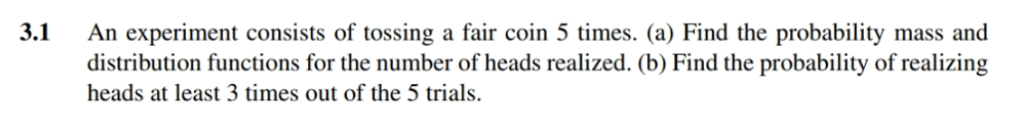a) here as number of trails are independent and probability of head on each event is fixed ; therefore it is binomial distribution with parameter n=5 and p=0.5

hence probability mass function of X:

P(X=x)=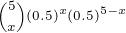frm above P(X=0)=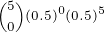=0.03125

P(X=1)=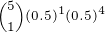=0.15625

P(X=2)=0.3125

P(X=3)=0.3125

P(X=4)=0.15625

P(X=5)=0.03125

probbaility distribution function F(x) of X is as follows:

 0 x<=0 0.03125 0<=x<1 0.1875 1<=x<2 F(x)= 0.5 2<=x<3 0.8125 3<=x<4 0.96875 4<=x<5 1 x>=5

b)

P(realizing at least 3 heads out of 5) =P(X=3)+P(X=4)+P(X=5) =0.3125+0.15625+0.03125=0.5

#### Earn Coins

Coins can be redeemed for fabulous gifts.

Similar Homework Help Questions
• ### An experiment consists of tossing a coin 14 times

An experiment consists of tossing a coin 14 times. (a) How many different outcomes are possible? (b) How many different outcomes have exactly 9 heads? (c) How many different outcomes have at least 2 heads? (d) How many different outcomes have at most 10 heads?

• ### Consider the experiment of tossing a fair coin four times. If we let X = the...

Consider the experiment of tossing a fair coin four times. If we let X = the number of times the coin landed on heads then X is a random variable. Find the expected value, variance, and standard deviation for X.

• ### An experiment consists of tossing a coin six times and observing the sequence of heads and...

An experiment consists of tossing a coin six times and observing the sequence of heads and tails. How many different outcomes have at least three tails?

• ### An experiment consists of tossing a fair coin (head H, and tail T) three times. The...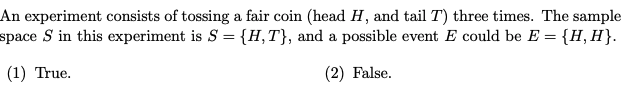An experiment consists of tossing a fair coin (head H, and tail T) three times. The sample space S in this experiment is S = {H, T}, and a possible event E could be E = {H,H}. (1) True. (2) False.

• ### 7. A random experiment consists of tossing a coin 4 times. Describe the sample space of...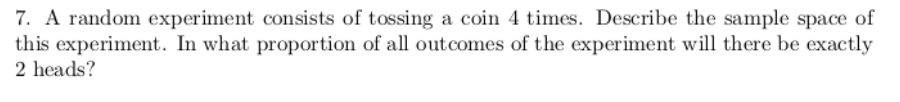7. A random experiment consists of tossing a coin 4 times. Describe the sample space of this experiment. In what proportion of all outcomes of the experiment will there be exactly 2 heads?

• ### An experiment consists in throwing a fair coin four times. Findthe pmf and the cdf of...

An experiment consists in throwing a fair coin four times. Findthe pmf and the cdf of the following random variables: (a)the number of heads minus the number of tails; (b)the number of heads times the number of tails.

• ### Find the probability of tossing exactly 2 heads on 3 tosses of a fair coin

Find the probability of tossing exactly 2 heads on 3 tosses of a fair coin.

• ### Example : In tossing a coin once , Find the probability of events ? 1) The...

Example : In tossing a coin once , Find the probability of events ? 1) The event A is the first tossing is heads 2) The event B is the first tossing is tails 3) The event c is the getting is one at least heads 4) The event c is the getting is one at most tails -------------------------------- Example : In tossing a coin once , Find the probability of events ? 1) The event A is the first...

• ### An experiment consists of tossing an unfair coin (53% chance of landing on heads) a specified...

An experiment consists of tossing an unfair coin (53% chance of landing on heads) a specified number of times and recording the outcomes. (a) What is the probability that the first head will occur on the second trial? (Use 4 decimal places.) Does this probability change if we toss the coin three times? What if we toss the coin four times? The probability changes if we toss the coin three times, but does not change if we toss the coin...

• ### Consider the experiment of simultaneously tossing a fair coin and rolling a fair die

Consider the experiment of simultaneously tossing a fair coin and rolling a fair die. Let X denote the number of heads showing on the coin and Y the number of spots showing on the die. a. List the outcomes in S.b. Find Fx,y(1,2).Part a is easy. It's part b that I don't understand. The answer given in the book is 4/12 but I don't see how they got that.